matlab函数 订阅
fzero，计算机用语，用来查找一元连续函数的零点。

fzero

x = fzero(fun,x0) %

matlab函数

：查找一元连续函数的零点。
fzero名称
matlab函数：fzero

• Matlab函数设置参数默认值
千次阅读
2021-05-06 01:18:56

再编写matlab函数时，通常有些参数会使用默认值，和其他语言不同，matlab有它自己的参数默认值设置方法。

在C++/C#/java/python等一系列的语言，甚至是R语言中，给函数设置默认值时都可以通过以下方式来设置：funtionName (param1, param2=defaultValue, ...)

注：一般含默认值的参数放到参数列表的后面。

在matlab中，这种方式便行不通了。可以通过以下两种方式给参数设置默认值，nargin是用来判断输入变量个数的函数，因此可通过判断参数个数来给默认参数赋值，更多nargin详细用法 在matlab窗口中输入help nargin查看帮助文档。

方式1 通过nargin来辅助实现(适用于1-2个较少默认值参数的函数)% 第三个参数有默认值

function [result]=funtionName (param1,param2,param3)

if (nargin<3)

param3 = defaultValue;

end

%函数其他操作

end

方式2 通过inputParser来实现function [result]=funtionName (varargin)

param= inputParser;    %接下来设定默认值，还可以指定是必须参数还是可选参数等。

%函数其他操作

%然后将输入的参数进行处理，如果有不同于默认值的那就覆盖掉

param.parse(varargin{:});

result=param.Results;

end

若想控制输出方式，可使用nargout输出参数来控制输出。综合实例如下function [x0, y0] = myplot(x, y, npts, angle, subdiv)

if nargin

if nargin

if nargin

...

if nargout == 0

plot(x, y)

else

x0 = x;

y0 = y;

end

end

参考文献：

MATLAB中nargin函数的用法：http://blog.sina.com.cn/s/blog_674956e70100j6om.html

【matlab】设定函数默认参数：https://www.cnblogs.com/zjutzz/p/5996161.html

更多相关内容
• ## MATLAB函数调用

千次阅读 2021-04-15 19:17:44
MATLAB函数调用 1、首先建立M函数文件右键——新建——函数，其中函数文件的格式、是： function [输出变量] = 函数名称(输入变量） 如下所示，是编写的一个求1到n之和的求和函数 A，按照上述格式，编写代码如下并...

## MATLAB函数调用

1、首先建立M函数文件右键——新建——函数，其中函数文件的格式是：
function [输出变量] = 函数名称(输入变量）
如下所示，是编写的一个求1到n之和的求和函数 A，按照上述格式，编写代码如下并保存文件，注意文件命名时不能以数字开头,且函数名需与文件名一致：

function n=A(n)
if n==1
n=1;
else
n=n+A(n-1)
end


2、然后使用保存的函数文件名来调用函数，依次把即将要被调用的文件保存到窗口Command Window运行的主界面文件夹，在Command Window窗口新起一行，写好文件名，把所有输入变量的具体数值填好，按键盘的回车键，即可表示调用运行了一次这个m文件得到的结果。
如下所示：

a=A(3)
或
A(3) %可直接输入


3、其次，在调用函数时注意要保存文件，且要将matlab当前目录切换到函数文件所在处

## 其他函数模板

1、当有多个形参时，形参之间用逗号分隔，形成形参表。

function y = A(x1,x2)
y1=x1+x2;
y2=x1-x2;
y=[y1,y2];
end


推荐：https://zhuanlan.zhihu.com/p/48627533

展开全文• ## Matlab函数求导教程

千次阅读 2021-04-18 03:46:03
Matlab是一款比较出名的商业数学软件，在这个软件，我们可以解答多种数学难题，但是对于刚接触这个软件的朋友来说，Matlab怎么进行函数求导是一个很大的难题，那么如果你不懂的话，赶紧看看小编整理的Matlab函数求导...

Matlab是一款比较出名的商业数学软件，在这个软件，我们可以解答多种数学难题，但是对于刚接触这个软件的朋友来说，Matlab怎么进行函数求导是一个很大的难题，那么如果你不懂的话，赶紧看看小编整理的Matlab函数求导教程吧！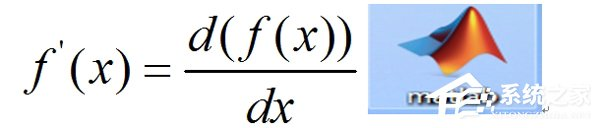方法/步骤：

1、diff(f(x))，一阶导数。

首先我们从最简单的一阶单变量函数进行求导，我们以f(x)=sin(x)+x^2 为例，进行示例介绍，在Matlab中，按照以下次序输入命令。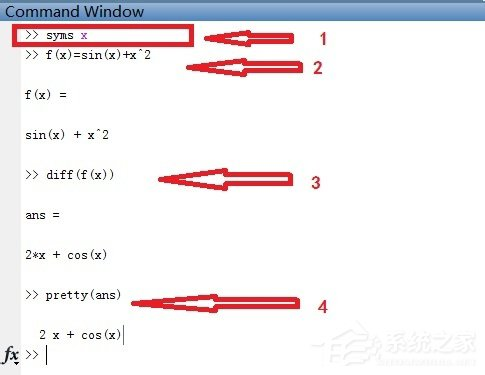说明：

(1)syms x代表着声明符号变量x，只有声明了符号变量才可以进行符号运算，包括求导。

(2)f(x)=sin(x)+x^2 ，就是我们输入的要求导的函数。

(3)diff(f(x))，代表着对单变量函数求一阶导数。

(4)pretty(ans)，将当前变量显示为我们常用的书面形式。

从以上结果我们可以看出，f(x)=2x+cos(x)。

2、diff(f(x)，n)，n阶导数。

我们还是以f(x)=sin(x)+x^2 为例，进行示例介绍，在Matlab中，按照以下次序输入命令。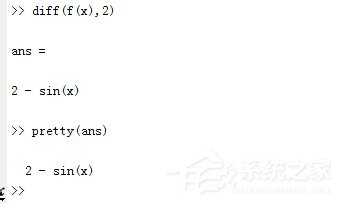可以看到，f(x)=2-sin(x)，N我们可以根据实际需要，取任意正整数。

3、diff(f(x1，x2，。。。。。)，xi)，对含有M个变量的函数求偏导数。

我们以函数f(x1，x2，x3)=sin(x1)+x2^2+exp(x3)为例进行介绍。

在Matlab主窗口中依次输入以下命令。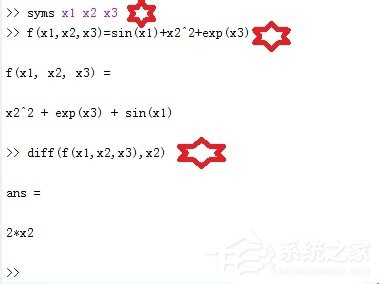我们可以看到，对于多元函数，我们需要将每一个变量声明为符号变量，如上，我们需要将x1，x2，x3声明为符号变量。然后diff(f(x1，x2，x3)，x2) 的意思是对x2求一阶偏导，结果为2*x2。

4、diff(f(x1，x2，。。。。。)，xi，N)，对多元函数的某一个变量求N阶偏导数。

我们还是以函数f(x1，x2，x3)=sin(x1)+x2^2+exp(x3)为例进行介绍。在MATLAB主窗口中依次输入以下命令。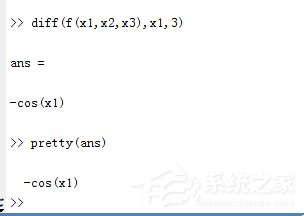我们可以看到，对x1进行求3阶数偏导数，可以看到sin(x1)的3阶导数为-cos(x1)。

以上就是Matlab函数求导的教程内容了，其中里面有介绍了一阶导数和n阶导数等的求导方法，大家可以进行尝试一下。

展开全文• Part 1Matlab函数的基本概念1.MATLAB的脚本和函数都是包含MATLAB命令的.m文件。2.脚本没有参数的输入输出，函数有。3.使用函数，需要一个脚本或者函数召唤它。1. MATLAB scripts and functions are .m files ...Part 1Matlab函数的基本概念

1.MATLAB的脚本和函数都是包含MATLAB命令的.m文件。

2.脚本没有参数的输入输出，函数有。

3.使用函数，需要一个脚本或者函数召唤它。

1. MATLAB scripts and functions are .m files containing MATLAB commands.

2. The script has no input and output parameters, but the function has.

3. To use a function, you need a script or function to call it.

Part 2查看内嵌函数

如查看平均数函数

Such as viewing the average function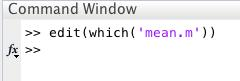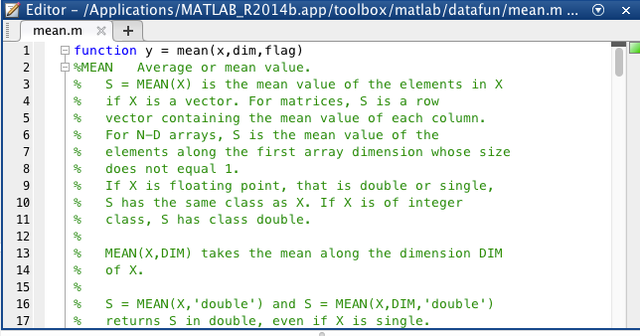通过上述内嵌函数，我们可以看到函数设置的五个要素：

Through the above embedded function, we can see the five elements of the function setting:

1.函数使用的关键字是function

2.文件名与函数名字是一致的

3.(x,dim,flag)是输入，y是输出

4.dim和flag为局部变量

5.MATLAB需要有找到该函数的路径

1. The keyword used by the function is function

2. The file name is consistent with the function name

3. (x, dim, flag) is input, y is output

4.dim and flag are local variables

5. MATLAB needs a path to find the function

Part 3用户自定义函数

如自定义自由落体位移函数

Such as custom free fall displacement function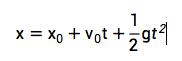首先新建脚本，输入函数，保存为与函数名相同名字的文件。

First create a new script, enter the function, and save it as a file with the same name as the function.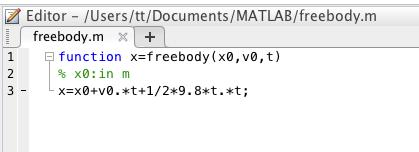注意：元素与元素之间是点乘。

然后就可以调用该函数了。

Note: There is a dot product between elements.

Then you can call the function.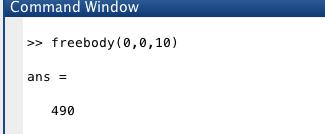调用内嵌函数和自定义函数是一致的。例如可以计算两组数据。

Calling built-in functions is consistent with custom functions. For example, two sets of data can be calculated.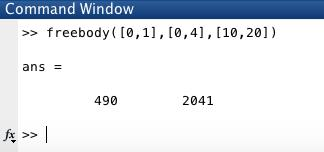Part 4多输入、多输出函数

如定义下列函数

Such as defining the following function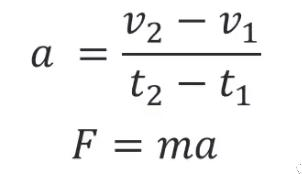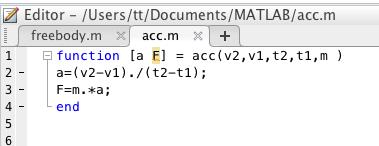然后调用函数Then call the function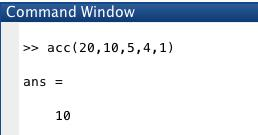我们会发现结果不对，多输入多输出函数的正确使用方法如下：

We will find that the result is wrong. The correct way to use the multiple-input multiple-output function is as follows: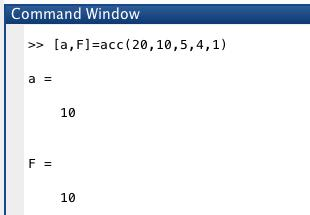Part 5没有input函数

例如，在命令栏输入一个华氏温度，然后计算成摄氏温度。

For example, enter a Fahrenheit temperature in the command bar, and then calculate it to Celsius.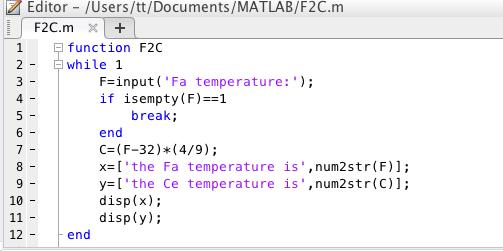Isempty:判断该函数是否为空

Num2str:将数值转化为字符串

然后运行程序，不停的输入，结果如下：

Isempty: Determine whether the function is empty

Num2str: Convert a value to a string

Then run the program, keep typing, the results are as follows: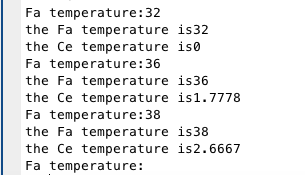参考资料：百度百科、B站郭彦甫Matlab教程、谷歌翻译

本文由LearningYard学苑原创，如有侵权，请联系删除。

举报/反馈

展开全文• MATLAB函数文件的使用 目录MATLAB函数文件的使用1.函数文件基本结构：（1）文件名（2）注释说明部分（3）函数体语句2.函数的调用2.1函数的递归调用3.函数参数的可调性4.局部变量和全局变量4.1局部变量4.2全局变量 1....
• matlab 函数返回多个值--样例发布时间：2018-02-14 19:52,浏览次数：2062, 标签：matlab目录一、matlab函数文件返回多个值写法二、简单的例子三、 结果截图一、matlab函数文件--返回多个值--写法为：1....
• ## MATLAB函数速查手册

万次阅读 多人点赞 2018-03-25 09:06:26matlab
• ## Matlab函数

千次阅读 2018-06-17 15:48:59
MATLAB中，函数在单独的文件中定义。文件的名称和函数的名称应该是一样的。函数在自己的工作空间内的变量上运行，这个变量也称为本地工作空间，与在MATLAB命令提示符下访问的工作区(称为基本工作区)不同。函数...
• ## MATLAB函数句柄

万次阅读 多人点赞 2019-05-15 10:44:51
感觉matlab函数句柄就是c语言里面的函数指针，在matlab里面叫它handle，句柄嘛，有了它就可以操纵这个对象(这里也可以叫做函数)，这个概念其实可以推广到很多东西，图形fig，自定义函数句柄(也就是下面将会展示的)，...
• 匿名用户1级2012-04-28 回答在Matlab7.0以后的版本中，出现了一种新的函数类型–匿名函数，不但能够完成原来版本中内联函数(inline)的功能，还提供了其他更方便的功能。Matlab首席科学家Moler教授都推荐用匿名函数...
• 本文研究Simulink模型中调用Matlab函数的方法。 文章目录1 Matlab Function模块2 建模与代码生成3 Matlab Function中的数据管理3.1 关联Signal对象3.2 建立函数内的参数4 Matlab Function生成代码的限制5 总结 1 ...
• 查看源码是学习写代码的一种好方法，这里介绍几种查看Matlab软件自带函数的方法：1、type函数在命令窗口输入“type+函数名”，则命令窗口会显示函数的源代码，例如type regress(其中regress是用来做线性回归的一个...
• C} if they're not going to be the same size, but then it won't display anyway end 或者如果你真的想要我猜你可以编写一个你在函数上调用的包装器函数,它会显示你可以在任何函数上使用的所有三个函数....
• 1、设置路径，将函数存储的文件夹放在可搜索路径下 2、函数文件名与函数名不同导致，此时使用的是函数文件名，而路径中没有改函数文件名
• 问题描述：MATLAB 函数先求导再赋值简单的说就是建立如下m文件function j=myfun(x)j=diff(x^2-1)进行如下调用s=myfun(2)得到s=[ ]也就是说在函数myfun调用时，是先赋值后求导的。而我希望得到先求导后赋值的结果s=4...
• ## MATLAB函数拟合使用

万次阅读 多人点赞 2019-06-07 18:37:56
1 函数命令拟合 最常用的函数拟合命令为fit，语法为| [拟合结果 拟合精度]＝fit（X数据，Y数据，‘拟合类型’） 其中，具体的拟合类型可以参看帮助文档，也可以使用fittype来自定义新的函数类型，比如定义拟合函数...
• ## MATLAB函数随笔画图篇

千次阅读 多人点赞 2020-07-18 10:03:20
这些都是我在学习MATLAB与数学建模时遇到的一些常用函数，就在这里写了下。当然MATLAB十分强大，函数众多，这些只是凤毛麟角，这篇博客我也会一直更新有新的函数就接着写上。有不足之处还望指出。
• 当求导的函数比较复杂，可以利用MATLAB中diff语句进行求解导数。 注：matlab中可以使用指令syms x定义一个符号变量x，符号变量可以进行算术运算、积分、求导等操作。 1、（一阶导数） syms x >> diff(x^...开发语言 算法 数学
• 关于Matlab的一些基本操作在另一篇博客里有提及 https://blog.csdn.net/Netceor/article/details/95043332 目录 想查找相关内容也可在页面按ctrl+F搜索文字 1.线性方程求解 2.非线性方程求解 3.多项式求解 4....
• 一、matlab函数文件返回一个值写法 二、简单的例子 三、结果截图 博主针对返回多个值也有相关样例，可见于博主文章：matlab 函数返回多个值--样例 一、Matlab函数文件--返回一个值--写法为： 1.第一行写 ...
• 我在文件中定义了两个函数，保存文件时Matlab会自动生成一个文件名，这个文件名一般是第一个函数名，如果我们最终执行的是第二个函数，运行的时候就会出现函数的参数错误。因此把最终执行的函数写在前面，保存自动...
• ## matlab函数定义

千次阅读 2019-06-11 14:26:22
matlab定义函数，和C语言类似，定义一个从华氏度到摄氏度的函数如下 function D =F2C() for i=0: 10 F = input('==>Temperature in F:','s');%input返回的是一个字符串，需要str2num转化成数字 if isempty(F) ...
• 1、函数功能 lower(s2)：将字符s转换为小写。 upper(s1)：将字符s转化为大写。 2、代码示例 clc; clear all; s1 = 'MaTlAB'; s = lower(s1) %将字符串s1转为小写 ss = upper(s1) %将字符串s1转为大写 输出...
• matlab的很多函数有着强大的功能，在python中调用matlab函数，无论是计算还是绘图都有巨大的前景，有时间会写写
• ## Matlab函数返回多个值--样例

万次阅读 多人点赞 2018-02-14 19:52:36
一、matlab函数文件返回多个值写法 二、简单的例子 三、结果截图 一、Matlab函数文件--返回多个值--写法为： 1.第一行写 function [返回值1/返回矩阵1，返回值2/返回矩阵2，...，返回值n/返回矩阵n]=该函数...
• 恰当地使用函数编写Matlab脚本可以提高MBD工作的自动化程度，提高工作效率。 本文会分类列举笔者工作中常用的函数，并简要说明其应用场景以及一些使用心得。同时也欢迎有其他项目经验的朋友在博客下留言补充。
• 这里就总结一下如何在VS工程中调用MATLAB函数，以及遇到的问题如何解决的方法。 VS配置 这一步很重要，类似于其他C++库的配置，如：添加包含目录和库路径。 添加库目录（自己的MATLAB安装路径中查找）：D:\...
• matlab函数返回值可以为一个结构体数组吗，如果能要怎么做...

# matlab函数matlab 订阅